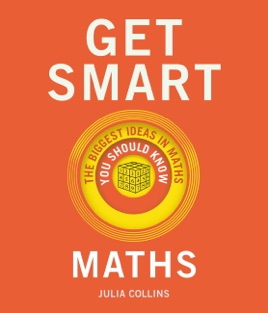• £3.99

## Publisher Description

Can you explain Fermat's Last Theorem? What is the shape of the Universe? And how do you add up to infinity?

Challenge yourself with Get Smart: Maths and learn to think and talk like the world's greatest mathematical geniuses.

Taking you on a journey through the mathematical ideas that underpin our world - from imaginary numbers and Turing machines to chaos theory and mathematical paradoxes; from the search for primes and game theory to relativity and the arithmetic of altruism - Get Smart: Maths demystifies 50 key concepts and provides you with the tools to master the very biggest ideas.

Includes: imaginary numbers; the riemann hypothesis; mathematical paradoxes; chaos theory; code breaking; Gödel's incompleteness theorem; topology; the Poincaré conjecture; game theory; the maths of symmetry; calculus; Turing machines; fractals; the prisoner's dilemma; primes; knot theory; probability and statistics; the Monty Hall problem . . . and many more.